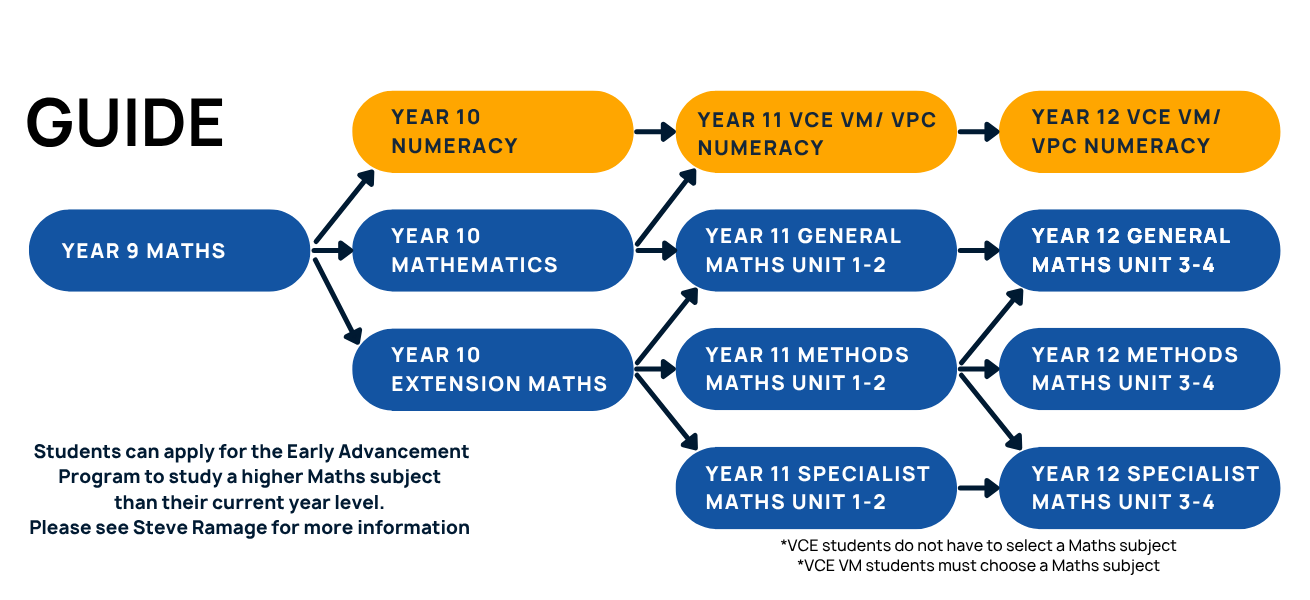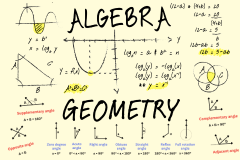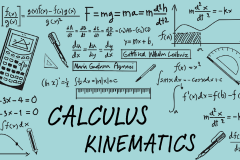## MATHEMATICS DOMAIN##### Steve Ramage
###### From its origins in counting and measuring, mathematics has evolved in highly sophisticated and elegant ways to become the language now used to describe many aspects of our world today. In the Mathematics Domain, we look to develop your mathematical and literacy skills for everyday life, work and assist you in becoming active and critical citizens in a technological world. You learn to see connections and apply mathematical concepts, skills and processes to pose and solve problems in mathematics and in a range of contexts. As you progress through your mathematical studies you are able to follow a pathway which is more suited to your needs, aspirations and interests.#### 9 Numeracy (VCAL Pathway)• Focus on mathematics that is useful in everyday life
• Cover topics such as measurement, financial mathematics and estimation
• Recommended for those who found Year 8 Standard Maths a challenge

Subject Code: P1MNU
Year Level: 9

##### What will I learn?

Apply real life mathematics here! Maths is needed in the community, at work, and in further study. Numeracy encompasses the knowledge, skills, behaviours and dispositions that students need to use mathematics in a wide range of situations in the real world.

Topics covered:

• Real life mathematics
• Estimation
• Measurement
• Financial Mathematics
• Statistics and Probability
• Graphing
##### What sort of student would like Numeracy?

Those who:

• Found it a challenge to meet the required standard for maths in Year 8, and for whom VCE mathematics is not a preferred option, or accessible.
• Would like to gain or consolidate basic ability.
• Want to follow a pathway into employment or further training. Suitable if you intend a VCAL or VCE pathway that does not include Maths in Year 11 and 12.
##### Where can this take me?

Subjects: 10 Numeracy, VCAL Numeracy.

2 semesters.

#### 9 Standard Mathematics• Learn practical skills that can be applied to the real world
• Cover topics such as Algebra, Geometry and Measurement
• Prepares students for mathematics in VCE

Subject Code: P1MST
Year Level: 9

##### What will I learn?

Continue to build on basic arithmetic skills in preparation for mathematics in the Senior School. Share mathematical ideas and have the opportunity to discuss mathematics with one another, refining and critiquing each other’s ideas and understandings.

Topics covered:

• Number
• Algebra
• Geometry
• Measurement
• Statistics and Probability
##### What sort of student would suit Standard Maths?

Those who:

• Met the required standard for Maths in Year 8. It is in line with Level 9 of the Victorian Curriculum.
• Intend to complete Maths at VCE level.
##### Where can this take me?

Subjects: 10 Pre-General, 10 Pre-Methods, 10 Numeracy.

2 semesters.

#### 10 Numeracy (VCAL Pathway)• Focus on mathematics that is useful in everyday life
• Cover topics such as measurement, financial mathematics and estimation
• Recommended for those who found Year 9 Standard Maths a challenge

Subject Code: P2MNU
Year Level: 10

##### What will I learn?

Apply real life mathematics here! Maths is needed in the community, at work, and in further study. Numeracy encompasses the knowledge, skills, behaviours and dispositions that students need to use mathematics in a wide range of situations in the real world.

Topics covered:

• Real life mathematics
• Estimation
• Measurement
• Financial Mathematics
• Statistics and Probability
• Graphing
##### What sort of student would like Numeracy?

Those who:

• Found it a challenge to meet the required standard for mathematics in Year 9, and for whom VCE mathematics is not a preferred option, or accessible.
•  Would like to gain or consolidate basic ability.
• Want to follow a pathway into employment or further training. Suitable if you intend a VCAL or VCE pathway that does not include Maths in Year 11 and 12.
##### Where can this take me?

Subjects: VCAL Numeracy

2 semesters.

#### 10 Pre-General• Explore the applications of mathematics in real life situations through a combination of group tasks and individual activities
• Focus on topics such as Financial Maths, Measurement and Trigonometry
• This prepares students for VCE General Mathematics Unit 1 & 2

Subject Code: P2MGE
Year Level: 10

##### What will I learn?

You develop basic problem solving required for General and Further Mathematics. Share mathematical ideas and have the opportunity to discuss mathematics with one another, refining and critiquing each other’s ideas and understandings. This class will prepare you for VCE General Mathematics Unit 1 and 2.

You are encouraged to use appropriate mathematical technology (Ti-nspire CAS calculator).

Topics covered:

• Linear Relations
• Financial Maths
• Measurement
• Trigonometry
• Networks
• Statistics
##### What sort of student would suit Pre-General Maths?

Those who:

• Understand the importance of Mathematics as part of your VCE pathway or if you enjoy aspects of Maths and are looking to keep career options open.
• Think you might need maths as a career pathways (trades, university degrees, retail.)
• A discussion with your Maths teacher and Learning Mentor can help decide the appropriate subject for you.
##### Where can this take me?

VCE Subjects: VCE General Mathematics, VCAL Numeracy

2 semesters.

##### Pre-requisites:

Any Year 9 Maths.

#### 10 Pre-Methods• Develop an understanding of the relationship between the ‘why’ and the ‘how’ of mathematics
• Focus on topics such as Algebra, Functions and Probability
• For students who enjoy the challenge of the harder algebraic based work

Subject Code: P2MME
Year Level: 10

##### What will I learn?

This is an introduction to VCE Mathematical Methods Unit 1 and 2. In this class you will demonstrate competency using directed numbers, algebra and operating with fractions. We develop abilities in calculator use (Ti-nspire CAS) and basic problem solving required for Mathematical Methods.

If you are unsure of what career to pursue, Mathematical Methods satisfies most paths.

Topics covered:

• Algebra
• Functions
• Probability
• Measurement
• Geometry
##### What sort of student would suit Pre-Methods?

Those who:

• Have a strong interest in mathematics, enjoy the challenge of the harder algebraic based work, and have a test average above 70% in Year 9 Maths or equivalent.

A discussion with your Maths teacher and Learning Mentor can help decide the appropriate unit for you.

Whilst it is possible to move from Mathematical Methods to General Mathematics, it is not possible to move the other way.

##### Where can this take me?

VCE Subjects: VCE General Mathematics, VCE Mathematical Methods

2 semesters.

##### Pre-requisites:

Year 9 Standard Maths. High achieving Year 8 students via application and interview.

#### Extension Mathematics• Combine experiments with modelling and analysis in mathematics
• Cover topics such as Calculus, Kinematics and Pythagoras Theorem

Subject Code: P3MEX
Year Level: 9 or 10

##### What will I learn?

We combine practical experiments with mathematical modelling and analysis. Experiments and activities include (but are not limited to) catapults, game shows, medical modelling and packaging design. A discussion with your current Mathematics teacher can help decide the most appropriate subject for you.

Topics covered:

• Optimisation/Calculus
• Kinematics
• Pythagoras' Theorem
• Probability
• Data Analysis
##### What sort of student would suit Extension Maths?

Someone who:

• Consider this subject if you enjoy and are strong at Mathematics and have a STEM career in mind.
• Have strong mathematical skills and find it stimulating and engaging to challenge themselves.

A discussion with your current Maths teacher can help decide the most appropriate subject for you.

##### Where can this take me?

VCE Subjects: VCE Specialist Mathematics, VCE Physics, VCE General Mathematics.

semester.

##### Pre-requisites:

Year 9 Standard Maths.